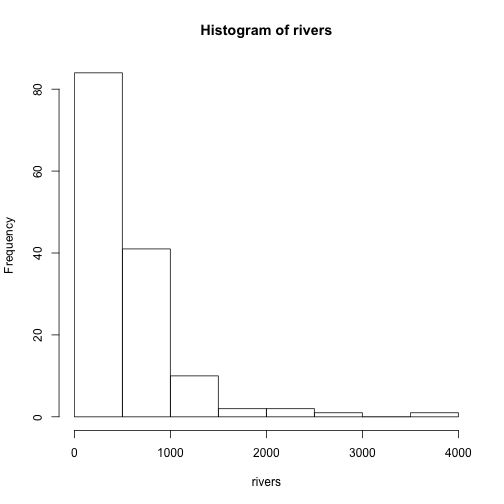# Lesson 1

The questions

This quiz uses JavaScript to be “interactive.” Unfortunately, it will only be so with browsers that are relatively modern, and this excludes Windows Internet Explorer 8 – the default browser on older installations of Windows. Please try and upgrade IE or install one of firefox or chrome to get the full benefits of this page.

This quiz covers some of the questions in the notes for week 1 of the R Intro Statistics Dot Com class. Please discuss on the online forum there if there are questions.

Enter the prime numbers 2,3,5,7,11,13,17 and 19 into a data vector called primes. There is no pattern here, just a collection of numbers.

Which command will work?

For the given value of a data set x:

x <- c(1,3,2,4,1,5,16,18)


Which command gives $$n$$, the number observations?

The range operator is very useful to define indices. Which of the following is produced by -1:5?

The “rule” of thumb for the standard normal has 99.7% of the area within 3 standard deviations of the mean (99.7 is $$0.997$$ on a proportiion scale). Find the exact amount using R (two 3 decimal points) (on the $$[0,1]$$ scale).

For the $$t$$-distribution with 8 degrees of freedom, find the value “tstar” when we want an 80% confidence interval.

There are many ways to access a variable in a list. Which of these is not a valid way to get the wt variable out of the mtcars data frame?

Which command will return the even-indexed values of:

x <- 1:10?


Which command will return the last element in the data set:

x <- c(2.6, 2.8, 2.3, 3.2, 3.4)


Which of these commands will subset the mtcars data set to get a new data set with cars whose miles per gallon is better than average?

This is a histogram of the built in rivers data set. Which command below will produce this?Run the commands to make a histogram. Is the data set symmetric or skewed?

library(MASS)
hist(Cars93\$MPG.highway)


A teacher wants to know if their English class is “better” than average (the national average). Suppose the mean SAT critical reading score was 497 with an unknown variance. The teachers scores were:

517 506 520 496 529 512 517 520 491 528


Find the $$p$$-value of a $$t$$-test of a one-sided alternative of greater.

The following measurements are from an experiment where the hypothesized mean would be 12. Run a $$t$$-test to find the $$p$$-value of a two-sided alternative.

x <- c(13.75, 12.34, 10.39, 12.04, 11.63, 12.38, NA, 12.09)


Which of the following is a 90% confidence interval for the mean of the tax variable of the Boston data set in the MASS package?